## “一个模型三个特征”实例剖析

“一个模型三个特征”这部分是理论知识，比较抽象，你看了之后可能还是有点懵，有种似懂非懂的感觉，没关系，这个很正常。接下来，我结合一个具体的动态规划问题，来给你详细解释。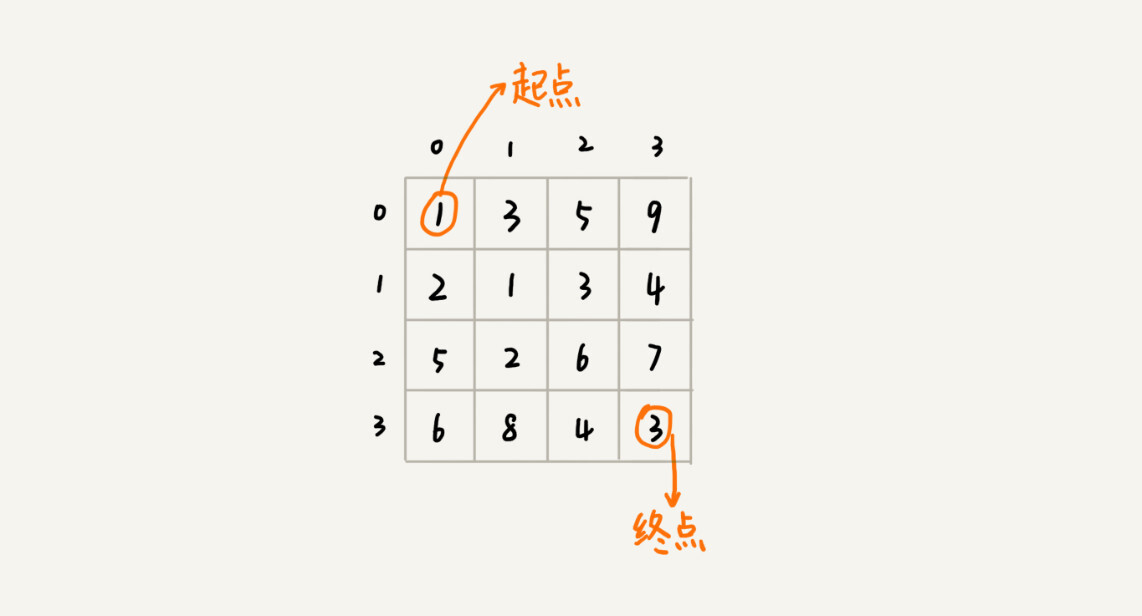(0, 0) 走到 (n-1, n-1)，总共要走 2*(n-1) 步，也就对应着 2*(n-1) 个阶段。每个阶段都有向右走或者向下走两种决策，并且每个阶段都会对应一个状态集合。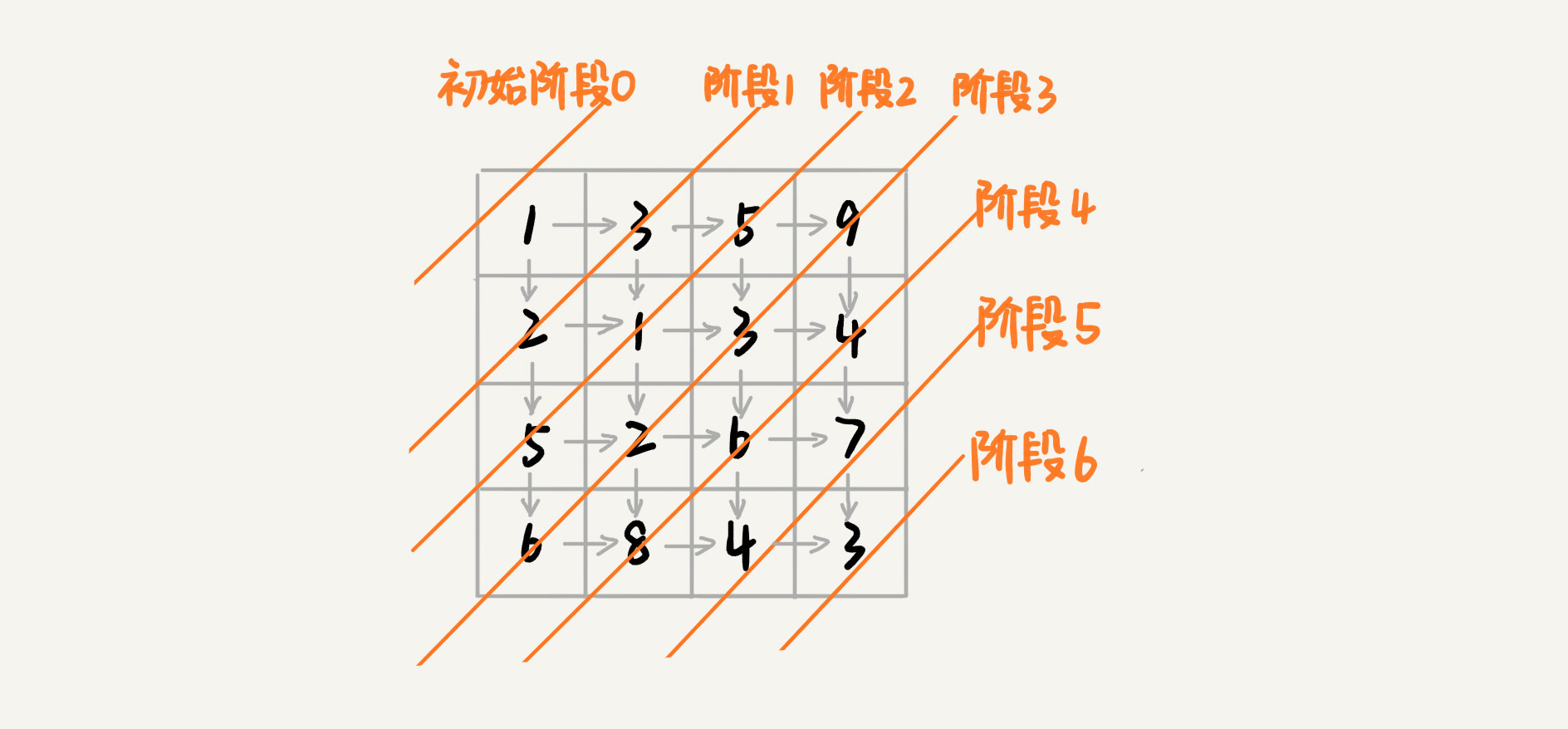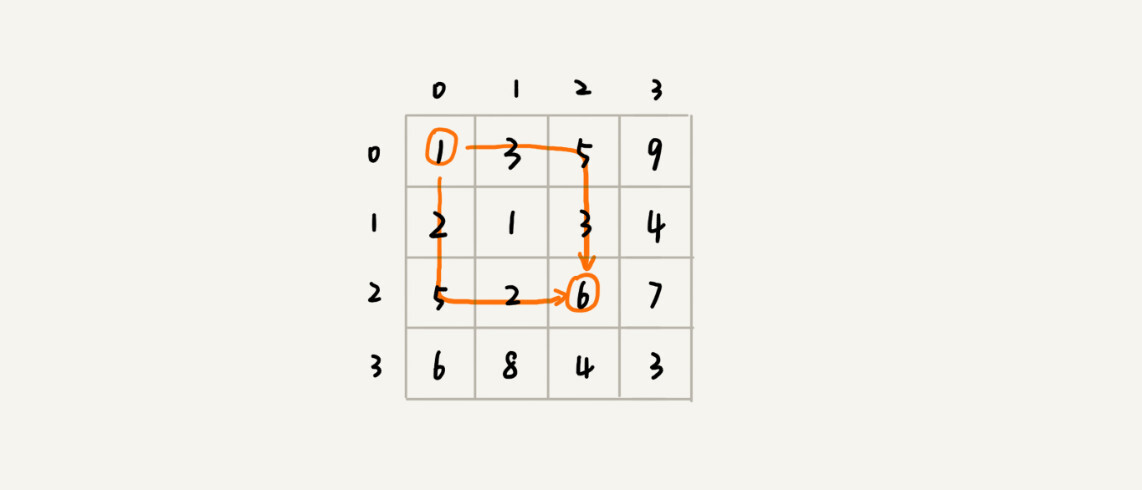`min_dist(i, j) = w[i][j] + min(min_dist(i, j-1), min_dist(i-1, j))`

## 两种动态规划解题思路总结

### 1. 状态转移表法

```private int minDist = Integer.MAX_VALUE; // 全局变量或者成员变量
// 调用方式：minDistBacktracing(0, 0, 0, w, n);
public void minDistBT(int i, int j, int dist, int[][] w, int n) {
// 到达了n-1, n-1这个位置了，这里看着有点奇怪哈，你自己举个例子看下
if (i == n && j == n) {
if (dist < minDist) minDist = dist;
return;
}
if (i < n) { // 往下走，更新i=i+1, j=j
minDistBT(i + 1, j, dist+w[i][j], w, n);
}
if (j < n) { // 往右走，更新i=i, j=j+1
minDistBT(i, j+1, dist+w[i][j], w, n);
}
}```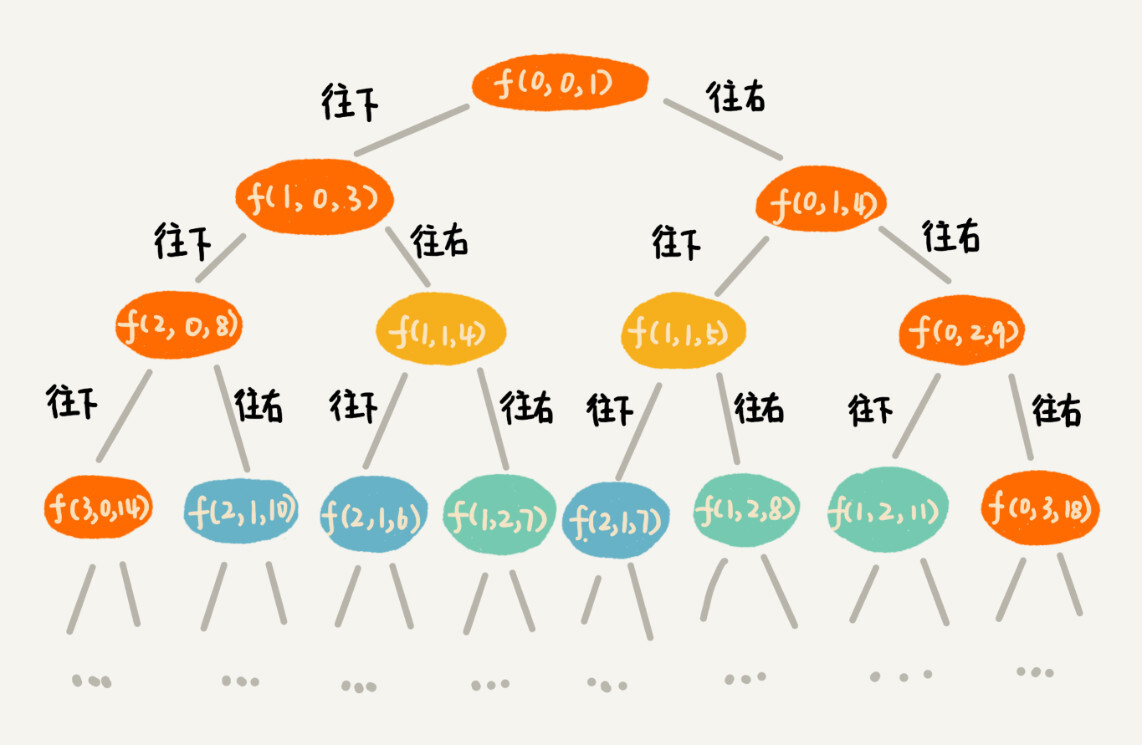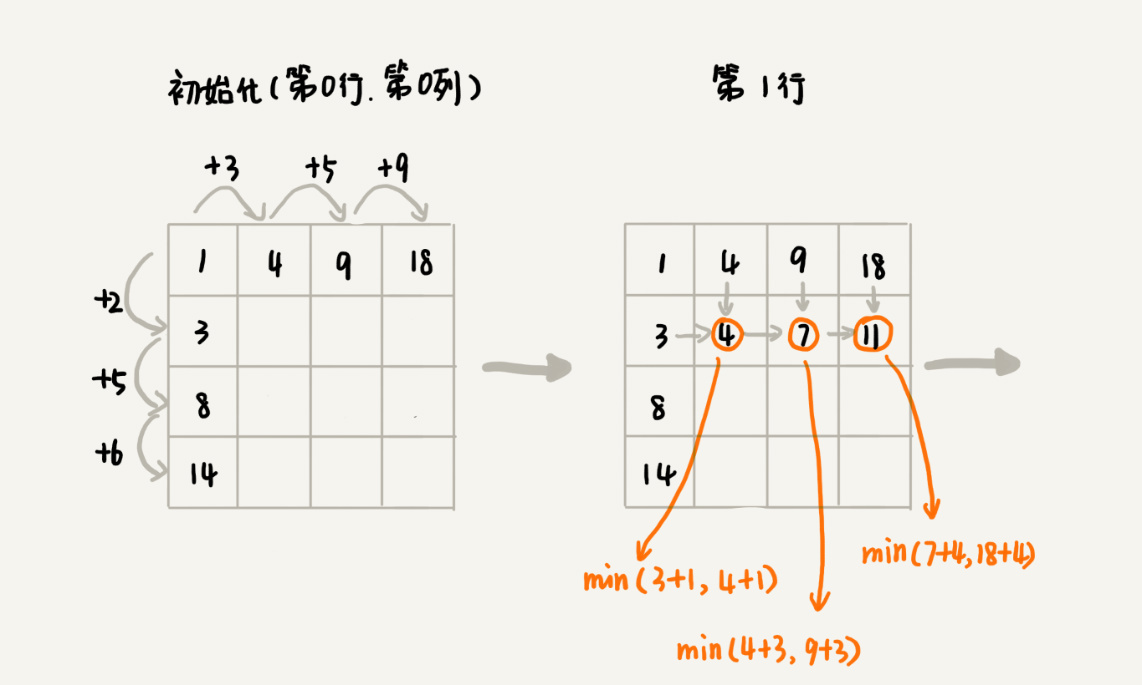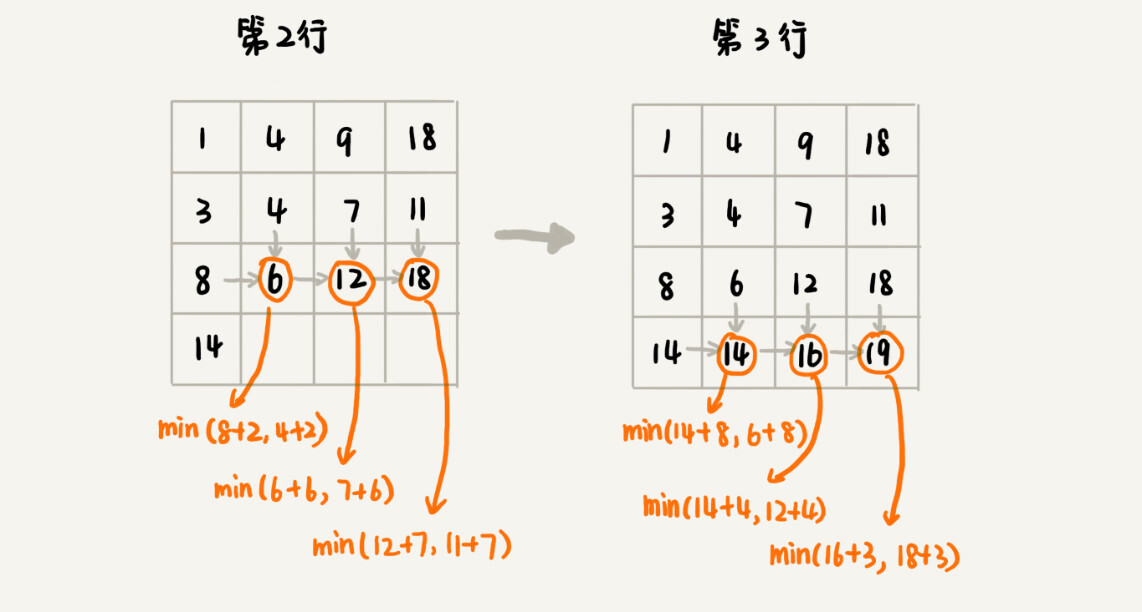```public int minDistDP(int[][] matrix, int n) {
int[][] states = new int[n][n];
int sum = 0;
for (int j = 0; j < n; ++j) { // 初始化states的第一行数据
sum += matrix[j];
states[j] = sum;
}
sum = 0;
for (int i = 0; i < n; ++i) { // 初始化states的第一列数据
sum += matrix[i];
states[i] = sum;
}
for (int i = 1; i < n; ++i) {
for (int j = 1; j < n; ++j) {
states[i][j] =
matrix[i][j] + Math.min(states[i][j-1], states[i-1][j]);
}
}
return states[n-1][n-1];
}```

### 2. 状态转移方程法

`min_dist(i, j) = w[i][j] + min(min_dist(i, j-1), min_dist(i-1, j))`

```private int[][] matrix =
{{1，3，5，9}, {2，1，3，4}，{5，2，6，7}，{6，8，4，3}};
private int n = 4;
private int[][] mem = new int;
public int minDist(int i, int j) { // 调用minDist(n-1, n-1);
if (i == 0 && j == 0) return matrix;
if (mem[i][j] > 0) return mem[i][j];
int minLeft = Integer.MAX_VALUE;
if (j-1 >= 0) {
minLeft = minDist(i, j-1);
}
int minUp = Integer.MAX_VALUE;
if (i-1 >= 0) {
minUp = minDist(i-1, j);
}

int currMinDist = matrix[i][j] + Math.min(minLeft, minUp);
mem[i][j] = currMinDist;
return currMinDist;
}```